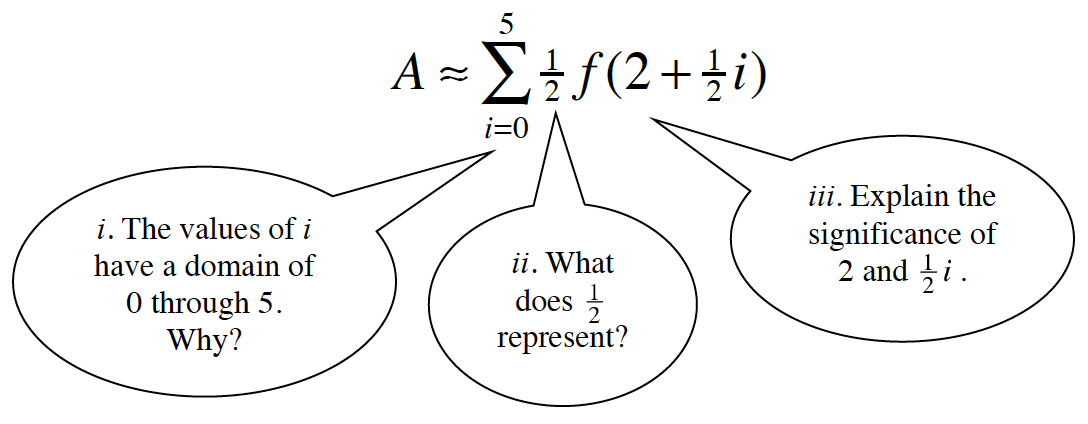### Home > APCALC > Chapter 2 > Lesson 2.1.3 > Problem2-40

2-40.

For the given function, write an expression using summation notation that will approximate the area under the curve for $3 \le x \le 7$ using eight rectangles. Specify if you use left, right, or midpoint rectangles. Then enter this summation expression into your graphing calculator and evaluate the approximate area.

$f(x) = \frac { 2 ( x + 4 ) } { x + 6 }$

Recall problem 2-17:i. For left-endpoint and midpoint rectangles, let the index start at $0$ and end at $n - 1$.
For right-endpoint rectangles, let the index start at $1$ and end at $n$.
$n = \text{number of rectangles}$.

$\text{ii. The }\frac{1}{2}\text{ represents the WIDTH/BASE of all rectangles.}$

To determine the width of each rectangle, divide the domain by the number of rectangles:

$\frac{7-3}{1}=\frac{1}{2}.$

$\text{Each rectangle has a width, }\Delta x \text{ is } \frac{1}{2}.$

iii. The transformed function represents the HEIGHT of each rectangle.
For left- and right-endpoint rectangles, the heights are represented by $f(a + \Delta xi)$.
For midpoint rectangles, the heights are represented by

$f \left(a+\frac{\Delta x}{2}i\right).$

$a = \text{starting value}$
$\Delta x = \text{width of each rectangle}$.